This is “Solving Linear Systems by Elimination”, section 4.3 from the book Beginning Algebra (v. 1.0). For details on it (including licensing), click here.

Has this book helped you? Consider passing it on:
Creative Commons supports free culture from music to education. Their licenses helped make this book available to you.
DonorsChoose.org helps people like you help teachers fund their classroom projects, from art supplies to books to calculators.

## 4.3 Solving Linear Systems by Elimination

### Learning Objectives

1. Solve linear systems using the elimination method.
2. Solve linear systems with fractions and decimals.
3. Identify the weaknesses and strengths of each method for solving linear systems.

## The Elimination Method

In this section, the goal is to develop another completely algebraic method for solving a system of linear equations. We begin by defining what it means to add equations together. In the following example, notice that if we add the expressions on both sides of the equal sign, we obtain another true statement.This is true in general: if A, B, C, and D are algebraic expressions, then we have the following addition property of equationsIf A, B, C, and D are algebraic expressions, where A = B and C = D, then A + C = B + D.:For the systemwe add the two equations together:The sum of y and −y is zero and that term is eliminated. This leaves us with a linear equation with one variable that can be easily solved:At this point, we have the x coordinate of the simultaneous solution, so all that is left to do is back substitute to find the corresponding y-value.Hence the solution to the system is (3, 2). This process describes the elimination (or addition) methodA means of solving a system by adding equivalent equations in such a way as to eliminate a variable. for solving linear systems. Of course, the variable is not always so easily eliminated. Typically, we have to find an equivalent system by applying the multiplication property of equality to one or both of the equations as a means to line up one of the variables to eliminate. The goal is to arrange that either the x terms or the y terms are opposites, so that when the equations are added, the terms eliminate. The steps for the elimination method are outlined in the following example.

Example 1: Solve by elimination: ${2x+y=73x−2y=−7$.

Solution:

Step 1: Multiply one, or both, of the equations to set up the elimination of one of the variables. In this example, we will eliminate the variable y by multiplying both sides of the first equation by 2. Take care to distribute.This leaves us with an equivalent system where the variable y is lined up to eliminate.Step 2: Add the equations together to eliminate one of the variables.Step 3: Solve for the remaining variable.Step 3: Back substitute into either equation or its equivalent equation.Step 4: Check. Remember that the solution must solve both of the original equations.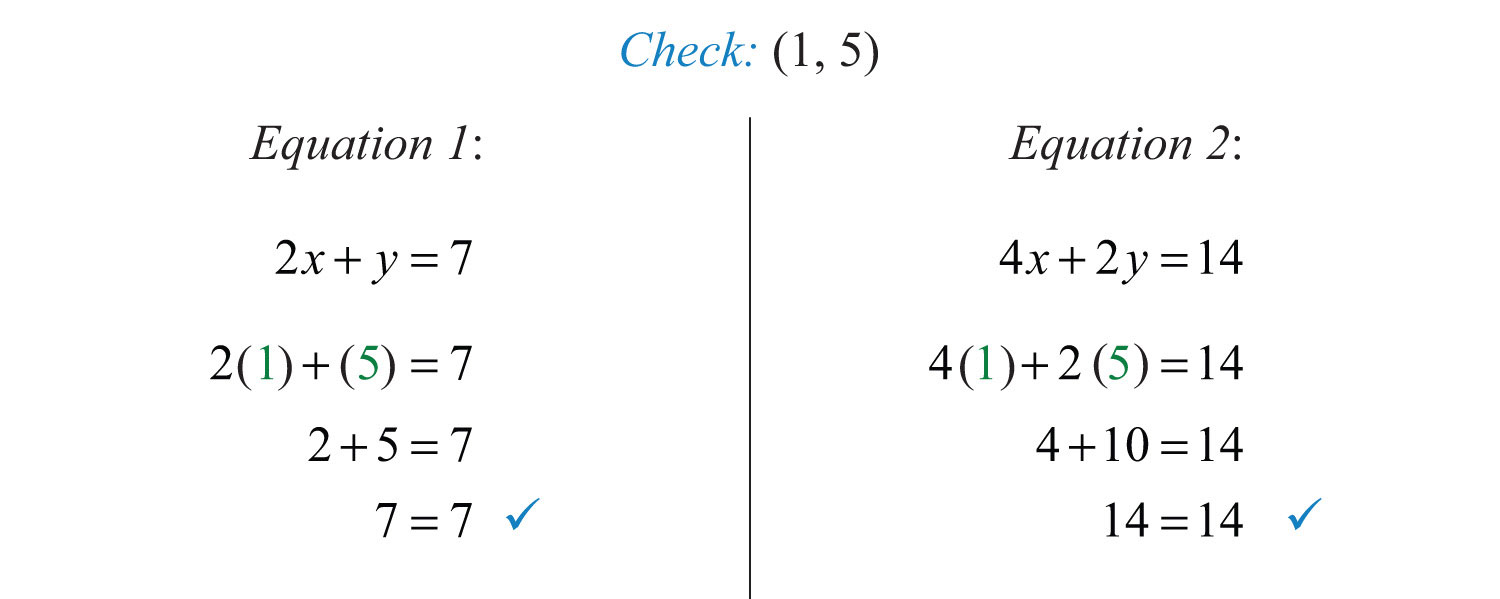Occasionally, we will have to multiply both equations to line up one of the variables to eliminate. We want the resulting equivalent equations to have terms with opposite coefficients.

Example 2: Solve by elimination: ${5x−3y=−13x+2y=7$.

Solution: We choose to eliminate the terms with variable y because the coefficients have different signs. To do this, we first determine the least common multiple of the coefficients; in this case, the LCM(3, 2) is 6. Therefore, multiply both sides of both equations by the appropriate values to obtain coefficients of −6 and 6.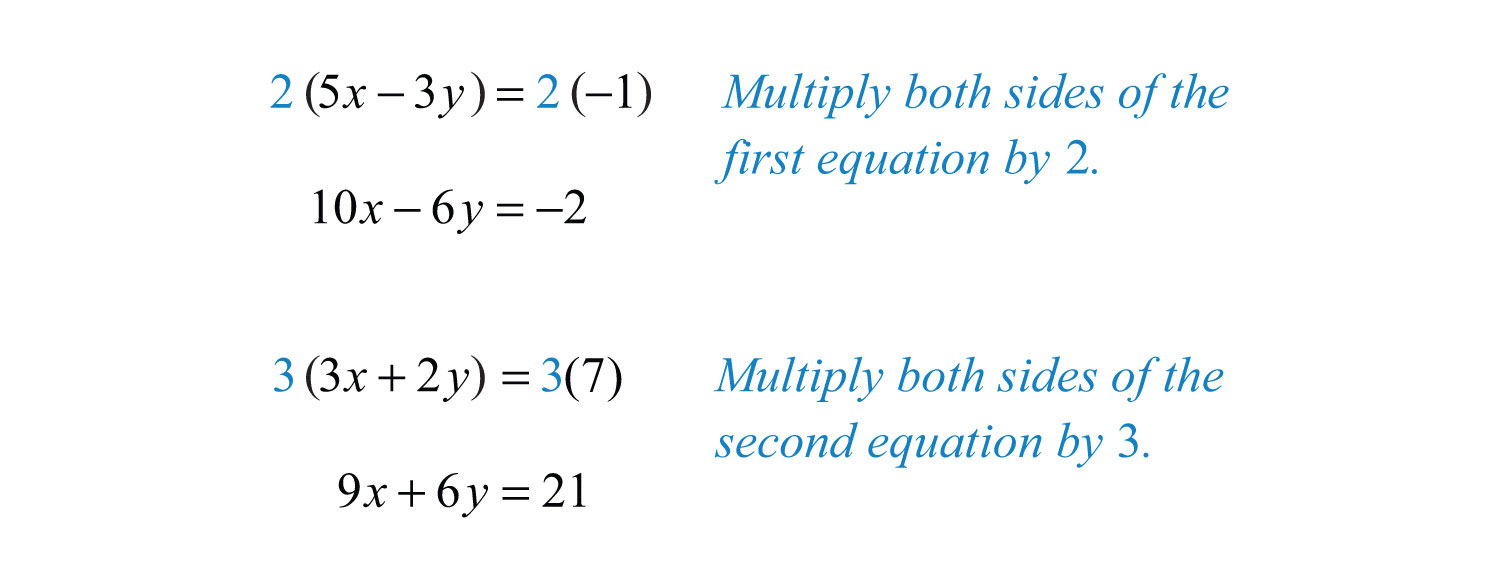This results in the following equivalent system:The y terms are now lined up to eliminate.Back substitute.Sometimes linear systems are not given in standard form. When this is the case, it is best to first rearrange the equations before beginning the steps to solve by elimination.

Example 3: Solve by elimination: ${5x+12y=113y=4x+1$.

Solution: First, rewrite the second equation in standard form.This results in the following equivalent system where like terms are aligned in columns:We can eliminate the term with variable y if we multiply the second equation by −4.Next, we add the equations together,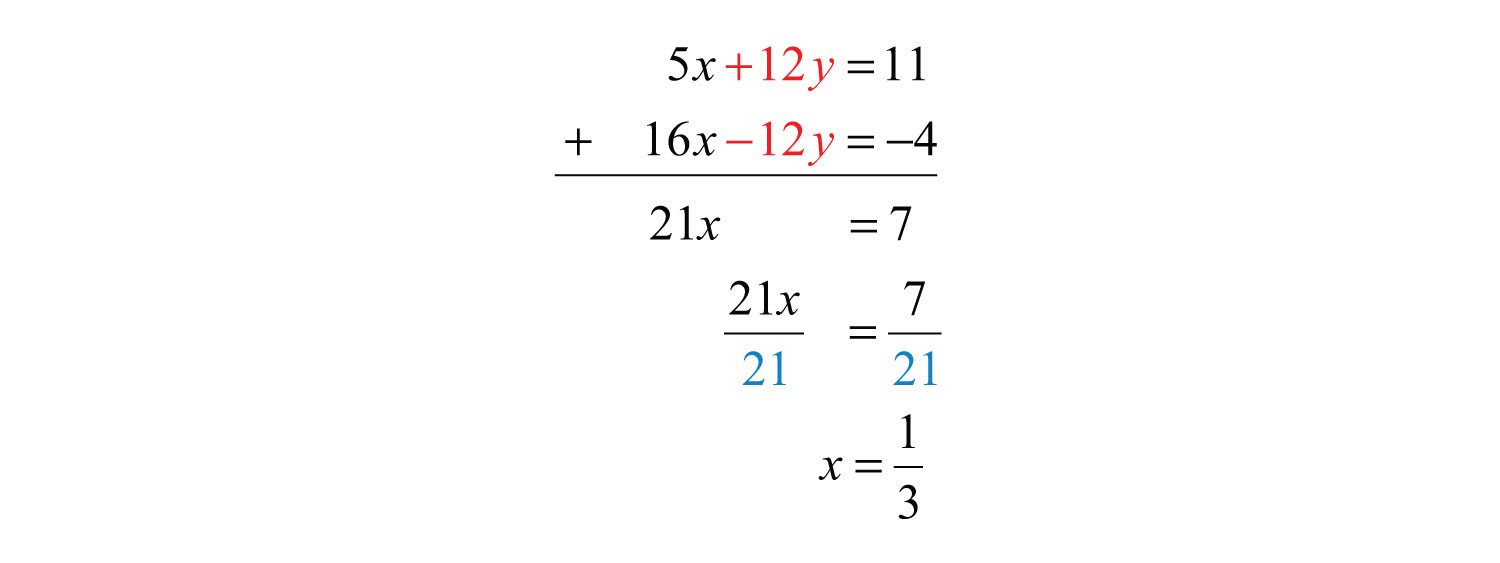Back substitute.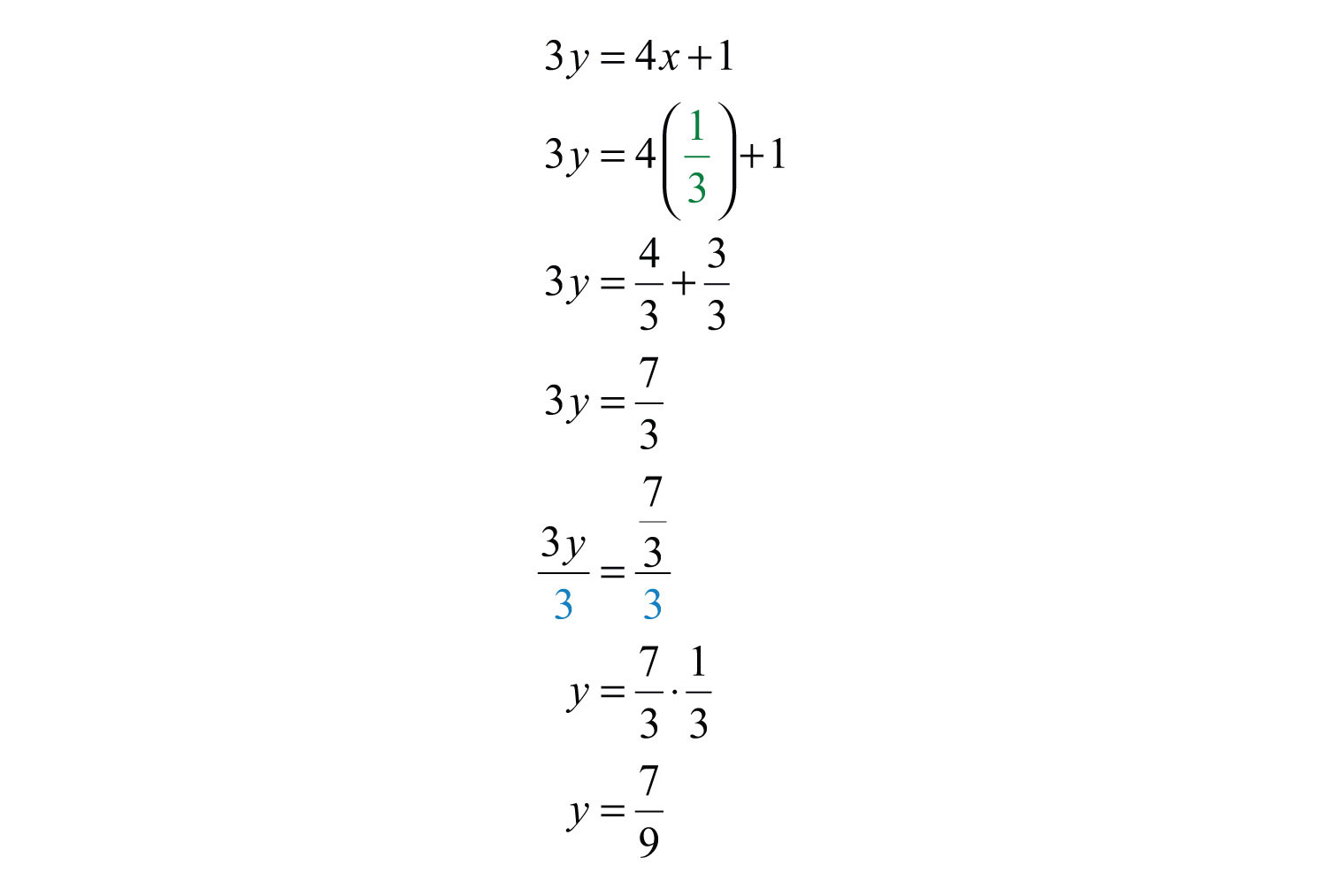Try this! Solve by elimination: ${2x+y=−3−3x−2y=4$.

### Video Solution

(click to see video)

At this point, we explore what happens when solving dependent and inconsistent systems using the elimination method.

Example 4: Solve by elimination: ${3x−y=76x−2y=14$.

Solution: To eliminate the variable x, we could multiply the first equation by −2.Now adding the equations we haveA true statement indicates that this is a dependent system. The lines coincide, and we need y in terms of x to present the solution set in the form $(x, mx+b)$. Choose one of the original equations and solve for y. Since the equations are equivalent, it does not matter which one we choose.Answer: $(x, 3x−7)$

Example 5: Solve by elimination: .

Solution: We can eliminate x by multiplying the first equation by 2.Now adding the equations we haveA false statement indicates that the system is inconsistent. The lines are parallel and do not intersect.

Answer: No solution, $∅$

Try this! Solve by elimination: ${3x+15y=−152x+10y=30$.

Answer: No solution, $∅$

### Video Solution

(click to see video)

## Clearing Fractions and Decimals

Given a linear system where the equations have fractional coefficients, it is usually best to clear the fractions before beginning the elimination method.

Example 6: Solve: .

Solution: Recall that we can clear fractions by multiplying both sides of an equation by the least common denominator (LCD). Take care to distribute and then simplify.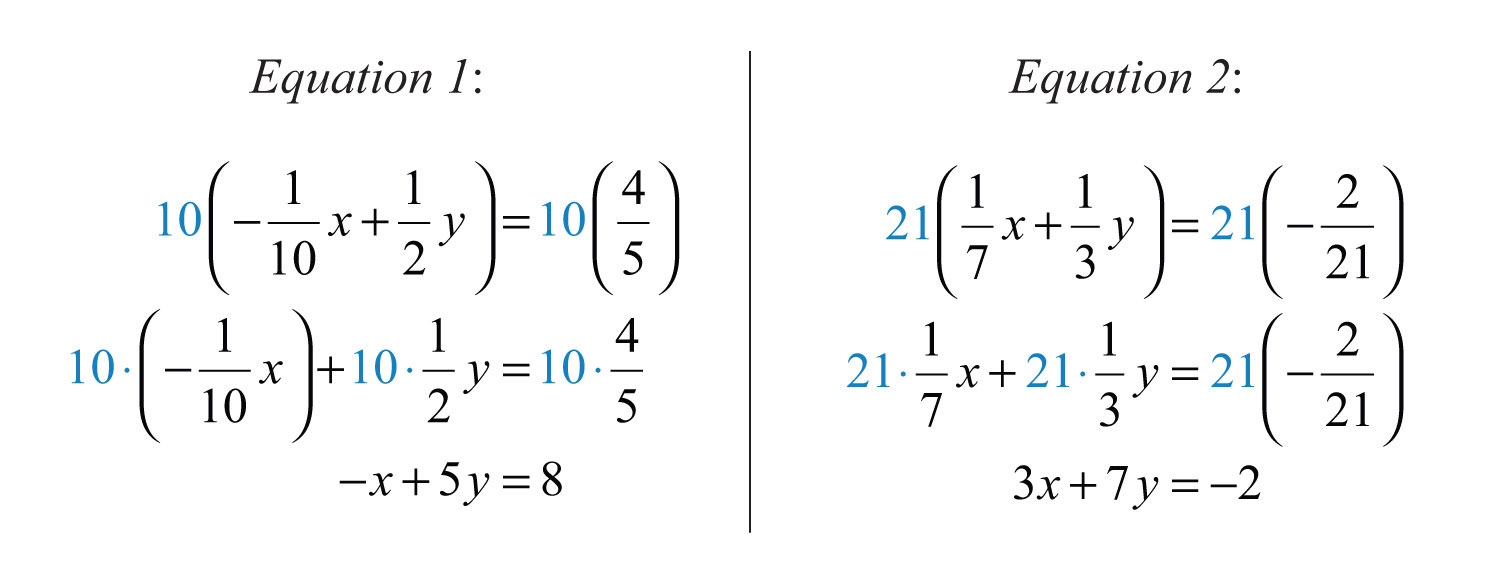This results in an equivalent system where the equations have integer coefficients,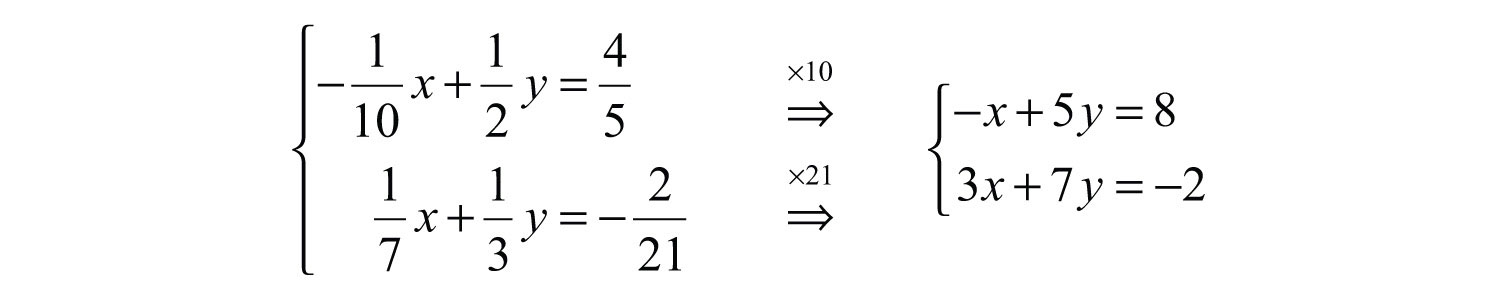Solve using the elimination method.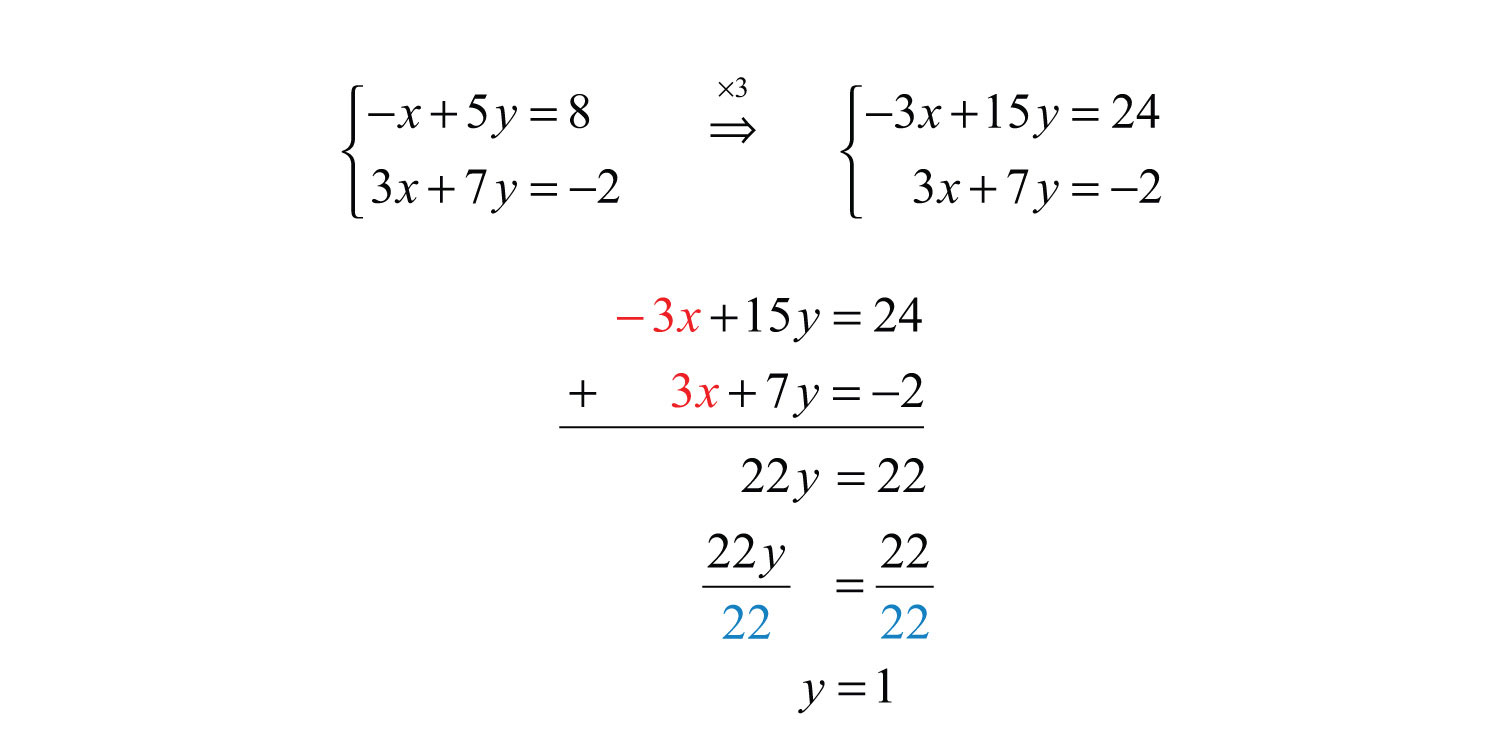Back substitute.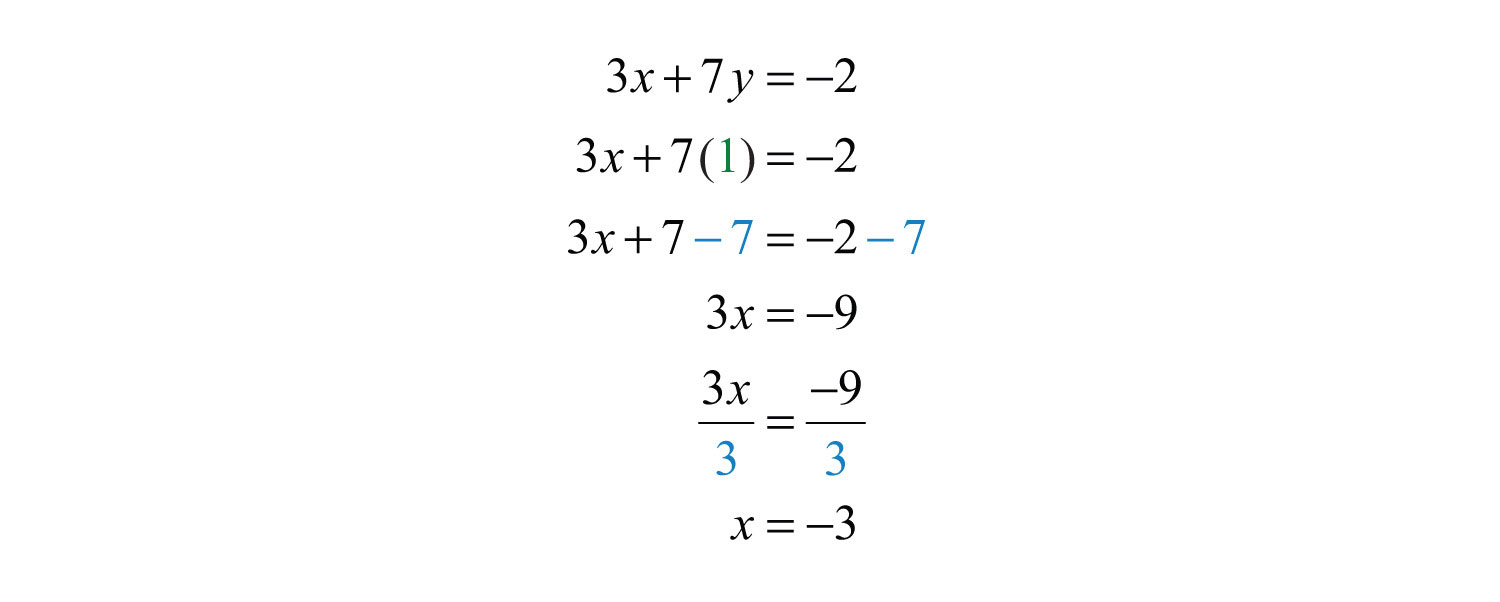We can use a similar technique to clear decimals before solving.

Example 7: Solve: ${3x−0.6y=−0.9−0.5x+0.12y=0.16$.

Solution: Multiply each equation by the lowest power of 10 necessary to result in integer coefficients. In this case, multiply the first equation by 10 and the second equation by 100.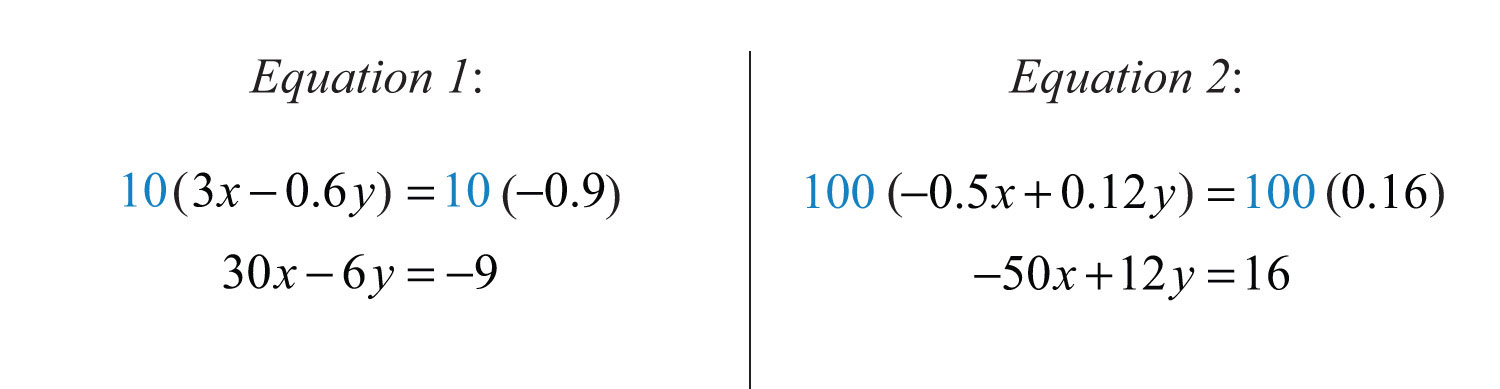This results in an equivalent system where the equations have integer coefficients:Solve using the elimination method.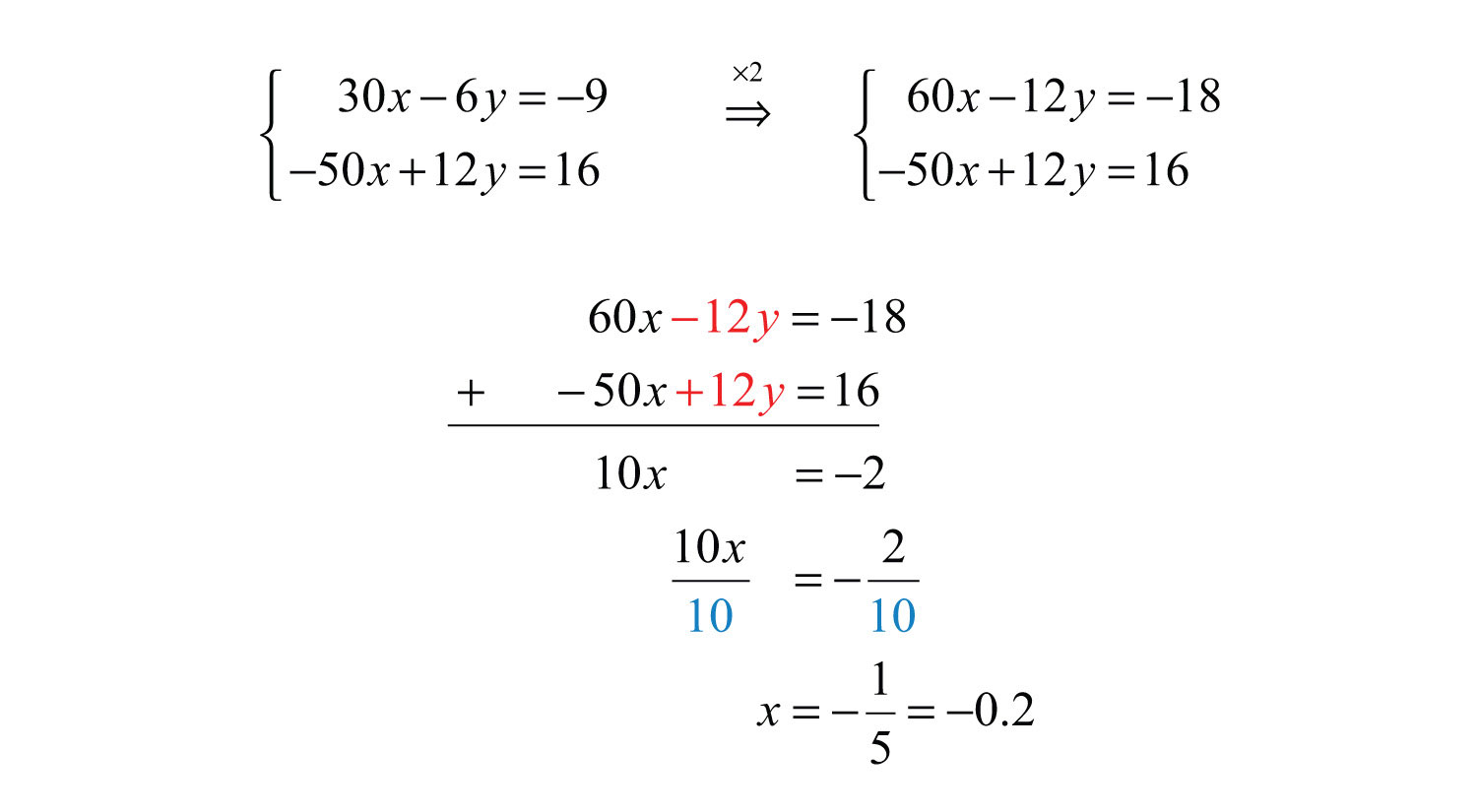Back substitute.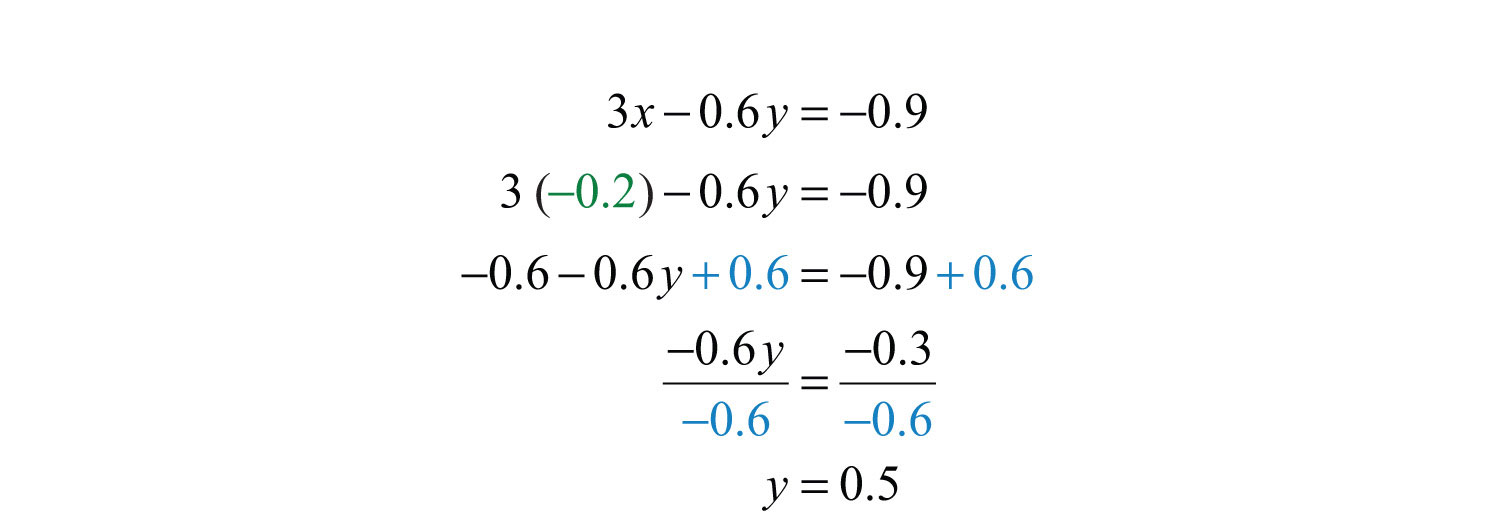Try this! Solve using elimination: ${13x−23y=313x−12y=83$.

### Video Solution

(click to see video)

## Summary of the Methods for Solving Linear Systems

We have developed three methods for solving linear systems of two equations with two variables. In this section, we summarize the strengths and weaknesses of each method.

The graphing method is useful for understanding what a system of equations is and what the solutions must look like. When the equations of a system are graphed on the same set of axes, we can see that the solution is the point where the graphs intersect. The graphing is made easy when the equations are in slope-intercept form. For example,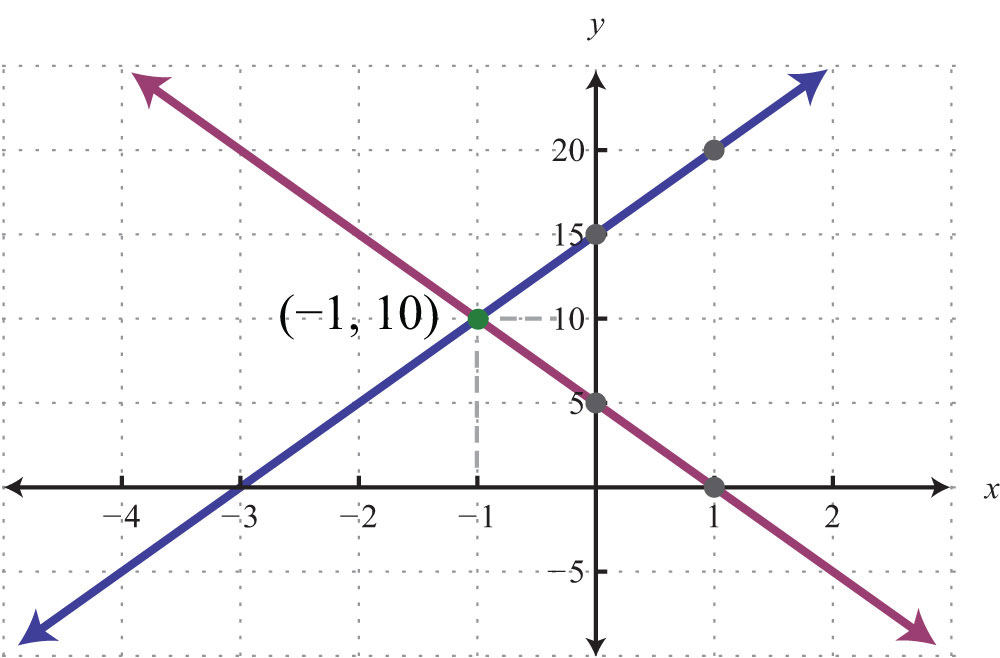The simultaneous solution (−1, 10) corresponds to the point of intersection. One drawback of this method is that it is very inaccurate. When the coordinates of the solution are not integers, the method is practically unusable. If we have a choice, we typically avoid this method in favor of the more accurate algebraic techniques.

The substitution method, on the other hand, is a completely algebraic method. It requires you to solve for one of the variables and substitute the result into the other equation. The resulting equation has one variable for which you can solve. This method is particularly useful when there is a variable within the system with coefficient of 1. For example,In this case, it is easy to solve for y in the first equation and then substitute the result into the other equation. One drawback of this method is that it often leads to equivalent equations with fractional coefficients, which are tedious to work with. If there is not a coefficient of 1, then it usually is best to choose the elimination method.

The elimination method is a completely algebraic method that makes use of the addition property of equations. We multiply one or both of the equations to obtain equivalent equations where one of the variables is eliminated if we add them together. For example,Here we multiply both sides of the first equation by 5 and both sides of the second equation by −2. This results in an equivalent system where the variable x is eliminated when we add the equations together. Of course, there are other combinations of numbers that achieve the same result. We could even choose to eliminate the variable y. No matter which variable is eliminated first, the solution will be the same. Note that the substitution method, in this case, would require tedious calculations with fractional coefficients. One weakness of the elimination method, as we will see later in our study of algebra, is that it does not always work for nonlinear systems.

### Key Takeaways

• The elimination method is a completely algebraic method for solving a system of equations.
• Multiply one or both of the equations in a system by certain numbers to obtain an equivalent system consisting of like terms with opposite coefficients. Adding these equivalent equations together eliminates a variable, and the resulting equation has one variable for which you can solve.
• It is a good practice to first rewrite the equations in standard form before beginning the elimination method.
• When the value of one of the variables is determined, back substitute into one of the original equations, or their equivalent equations, and determine the corresponding value of the other variable.

### Topic Exercises

Part A: Elimination Method

Solve by elimination.

1. ${x+y=32x−y=9$

2. ${x−y=−65x+y=−18$

3. ${x+3y=5−x−2y=0$

4. ${−x+4y=4x−y=−7$

5. ${−x+y=2x−y=−3$

6. ${3x−y=−26x+4y=2$

7. ${5x+2y=−310x−y=4$

8. ${−2x+14y=28x−7y=21$

9. ${−2x+y=412x−6y=−24$

10. ${x+8y=33x+12y=6$

11. ${2x−3y=154x+10y=14$

12. ${4x+3y=−103x−9y=15$

13. ${−4x−5y=−38x+3y=−15$

14. ${−2x+7y=564x−2y=−112$

15. ${−9x−15y=−153x+5y=−10$

16. ${6x−7y=42x+6y=−7$

17. ${4x+2y=4−5x−3y=−7$

18. ${5x−3y=−13x+2y=7$

19. ${7x+3y=92x+5y=−14$

20. ${9x−3y=37x+2y=−15$

21. ${5x−3y=−7−7x+6y=11$

22. ${2x+9y=83x+7y=−1$

23. ${2x+2y=53x+3y=−5$

24. ${−3x+6y=−122x−4y=8$

25. ${25x+15y=−115x+10y=−1$

26. ${2x−3y=218x−12y=5$

27. ${y=−2x−3−3x−2y=4$

28. ${28x+6y=96y=4x−15$

29. ${y=5x+15y=−5x+5$

30. ${2x−3y=95x−8y=−16$

31. ${12x−13y=1652x+y=72$

32. ${14x−19y=1x+y=34$

33. ${12x−14y=1314x+12y=−196$

34. ${−143x+2y=4−13x+17y=421$

35. ${0.025x+0.1y=0.50.11x+0.04y=−0.2$

36. ${1.3x+0.1y=0.350.5x+y=−2.75$

37. ${x+y=50.02x+0.03y=0.125$

38. ${x+y=300.05x+0.1y=2.4$

Set up a linear system and solve it using the elimination method.

39. The sum of two numbers is 14. The larger number is 1 less than two times the smaller.

40. The sum of two numbers is 30. The larger is 2 more than three times the smaller.

41. The difference of two numbers is 13 and their sum is 11.

42. The difference of two numbers is 2 and their sum is −12.

Part B: Mixed Exercises

Solve using any method.

43. ${y=2x−33x+y=12$

44. ${x+3y=−5y=13x+5$

45. ${x=−1y=3$

46. ${y=12x+9=0$

47. ${y=x−x+y=1$

48. ${y=5xy=−10$

49. ${3y=2x−243x+4y=2$

50. ${y=−32x+1−2y+2=3x$

51. ${7y=−2x−17x=2y+23$

52. ${5x+9y−14=03x+2y−5=0$

53. ${y=−5 16x+10y=5 16x−10$

54. ${y=−65x+12x=6$

55. ${2( x−3)+y=03( 2x+y−1)=15$

56. ${3−2( x−y)=−34x−3( y+1)=8$

57. ${2( x+1)=3( 2y−1)−213( x+2)=1−(3y−2)$

58. ${x2−y3=−7x3−y2=−8$

59. ${x4−y2=34x3+y6=16$

60. ${13x−23y=313x−12y=83$

61. ${−1 10x+12y=4517x+13y=−2 21$

62. ${y=−53x+1213x+15y=1 10$

63. ${−17x+y=−23−1 14x+12y=13$

64. ${1 15x−1 12y=13−3 10x+38y=−32$

65. ${x+y=4,2000.03x+0.0525y=193.5$

66. ${x+y=3500.2x+0.1y=52.5$

67. ${0.2x−0.05y=0.430.3x+0.1y=−0.3$

68. ${0.1x+0.3y=0.30.05x−0.5y=−0.63$

69. ${0.15x−0.25y=−0.3−0.75x+1.25y=−4$

70. ${−0.15x+1.25y=0.4−0.03x+0.25y=0.08$

Part C: Discussion Board Topics

71. How do we choose the best method for solving a linear system?

72. What does it mean for a system to be dependent? How can we tell if a given system is dependent?

1: (4, −1)

3: (−10, 5)

5: $∅$

7: (1/5, −2)

9: $(x, 2x+4)$

11: (6, −1)

13: (−3, 3)

15: $∅$

17: (−1, 4)

19: (3, −4)

21: (−1, 2/3)

23: $∅$

25: (1/5, −2/5)

27: (−2, 1)

29: (−1, 10)

31: (1, 1)

33: (−2, −16/3)

35: (−4, 6)

37: (2.5, 2.5)

39: The two numbers are 5 and 9.

41: The two numbers are 12 and −1.

43: (3, 3)

45: (−1, 3)

47: Ø

49: (6, −4)

51: (3, −1)

53: (32, 0)

55: $(x, −2x+6)$

57: (−4, 3)

59: (1, −1)

61: (−3, 1)

63: $∅$

65: (1,200, 3,000)

67: (0.8, −5.4)

69: Ø